• 绘制密度分布 y=rand(100,1)*10;%产生0-10以内的100个随机数 x=linspace(min(x),max(x),10);%产生10个等距数列 yy=hist(y,x);%统计分别落在10个等距区间的数量 %hist(y,20); %绘制20个等间距内数量的直方图 yy=yy/...
% 1.绘制密度分布 y=rand(100,1)*10;%产生0-10以内的100个随机数 x=linspace(min(x),max(x),10);%产生10个等距数列 yy=hist(y,x);%统计分别落在10个等距区间的数量 %hist(y,20); %绘制20个等间距内数量的直方图 yy=yy/length(x);%统计每个区间内占比例，即概率 bar(x,yy);%绘制直方图
%2. 核函数平滑函数估计 产生平滑的概率密度函数 x=10+0.1*randn(100,1);%产生100个符合均值10，标准差0.1d的正态分布的数列 [f, xi] = ksdensity(x);%核函数平滑函数估计.概率密度估计f plot(xi, f)
%3. 广义极值分布函数Generalized Extreme Value distribution(GEV)     %极值分布是指在概率论中极大值（或者极小值）的概率分布，从很多个彼此     %独立的值中挑出来的各个极大值应当服从的概率密度分布数f(x)。 clc;clear; k=0.1;%形状shape参数 sigma=5;%比例scale参数 mu=20;%均值loacation参数 R = gevrnd(k,sigma,mu,100,1);%产生指定参数的GEV随机数列 hist(R)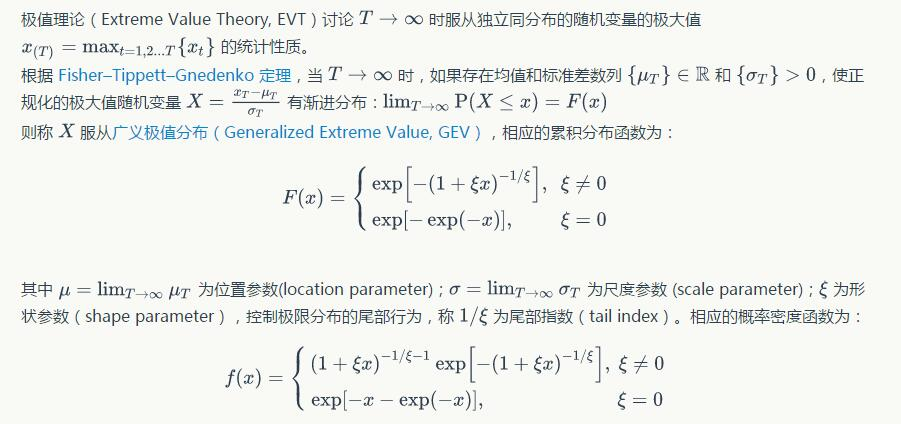展开全文• Matlab中Ksdensity()函数的用途：（画概率密度函数Matlab中Ksdensity()函数的用途：（画概率密度函数） http://blog.sina.com.cn/s/blog_6399df820100s8md.html [f,xi] = ksdensity(x) 计算样本向量x的概率密度...
Matlab中Ksdensity()函数的用途：（画概率密度函数）

Matlab中Ksdensity()函数的用途：（画概率密度函数） http://blog.sina.com.cn/s/blog_6399df820100s8md.html

[f,xi] = ksdensity(x)
计算样本向量x的概率密度估计，返回在xi点的概率密度f，此时我们使用plot(xi,f)就可以绘制出概率密度曲线。该函数，首先统计样本x在各个区间的概率(与hist有些相似)，再自动选择xi，计算对应的xi点的概率密度
展开全文• 函数绘制数据 X 的估计概率密度（核平滑），带宽为 b 并指示 n 个极值。 该设计遵循 Rahmstorf 等人。 在 Spektrum der Wissenschaft 中。 适用于例如显示时间序列分布中的极端年份。 请随意修改它（例如颜色图等...
• seid=16338053070486680597&...%%绘制直方图+概率密度函数+累积分布函数 %矩阵A是random生成0-1的随机数，或读入自己的数据 A = rand(1,500)*50; %求A的最大、最小、均值 maxA = max(A); minA = min(A); meanA = mean
视频学习：https://www.bilibili.com/video/BV1HK411T76d?from=search&seid=16338053070486680597&spm_id_from=333.337.0.0
总结如下：
%%绘制直方图+概率密度函数+累积分布函数
%矩阵A是random生成0-1的随机数，或读入自己的数据
A = rand(1,500)*50;

%求A的最大、最小、均值
maxA = max(A);
minA = min(A);
meanA = mean(A);
%求极差、中位数、偏度、峰度
RangeA = range(A);
medianA = median(A);
skewnessA = skewness(A);
kurtosisA = kurtosis(A);

%生成一个画布
pic = figure;
%绘制直方图(A中的元素有序划分入 x 轴上介于A的最小值和最大值间的 50 个等间距 bin中),centers表示每个bin中心在x轴
[counts,centers] = hist(A,50);
%绘制条形图，counts / sum(counts)计算频率
pic1 = bar(centers,counts / sum(counts),1);

set(pic1,'FaceColor','y')%设置矩形框颜色，‘b’为蓝色
set(pic1,'BarWidth',1);%设置矩形框宽度
%gcf返回当前axis对象的句柄值（一幅图像的每一个组成部分是一个对象）
%gca返回当前Figure对象的句柄值
set(gcf,'position',[220,200,900,600]);%设置画布的位置（220，200）和大小（宽900高600）
set(gcf,'position',[0.1,0.1,0.8,0.8]);%调整图形的位置和比例

axis([0 50 0 0.035]);%设置坐标轴范围[xmin xmax ymin ymax]

%计算概率密度函数,返回在xi点的概率密度f
[f,xi] = ksdensity(A);
hold on   %保留当前figure,实现图层叠加
pic2 = plot(xi,f,'LineWidth',1,'LineStyle','-','color','K');%绘制概率密度曲线

%计算概率密度积分分布函数
one_A = unique(A); %unique返回不重复的元素，产生的结果按升序排序

n_elements = histc(A,one_A);%统计在给定区间内的值的个数，左闭右开

c_elements = cumsum(n_elements);%计算元素累加的函数
%AX是坐标轴的句柄，AX（1）是左边的纵轴，AX（2）是右边的纵轴，H1,H2保存的是图形句柄，即两条线
[AX,H1,H2] = plotyy(0,0,one_A,c_elements);
%设置坐标轴的属性
set(AX(1),'ytick',0:0.005:0.035);%设置左边纵轴的刻度
set(AX(2),'ytick',0:50:500);%设置右边纵轴的刻度
set(AX(1),'ylim',[0,0.035]);%设置左边纵轴的取值范围
set(AX(2),'ylim',[0,500]);%设置右边纵轴的取值范围
%设置累积分布函数的线宽
set(H1,'LineWidth',2,'LineStyle','-','color',[0.9400 0.9400 0.9400]);
set(H2,'LineWidth',2)
%设置概率密度函数的线颜色
set(pic2,'LineWidth',2,'color','b');
%设置轴的颜色
set(AX(1),'ycolor','b');

set(AX(1),'ygrid','on');%打开左边纵轴网格
set(AX(2),'ygrid','on');%打开右边纵轴网格
%set(AX(1),'ygrid','off');%关闭左边纵轴网格

%设置title
title('test PDF')
%获取title的相关属性:get(gca,'title')
%设置字符、字体大小
set(get(gca,'title'),'String','Test PDF','FontSize',14);
set(get(gca,'title'),'Position',[25.001 0.0355 0]);%调整title位置

xlabel('X')%设置横轴标签
%set(get(gca,'xlabel'),'String','FontSize',14);%设置字体大小
set(get(AX(1),'ylabel'),'String','PDF','FontSize',14);%设置左纵轴标签
set(get(AX(2),'ylabel'),'String','CDF','FontSize',14);%设置右纵轴标签
set(get(AX(2),'ylabel'),'String','CDF','rotation',270,'Position',[77 250.0002-1.0000]);%设置旋转角度,标签位置

%添加文本 text(x,y,'')
textA = text(-10,0.025,{['Range:',num2str(RangeA)];['Max:',num2str(maxA)];['Mean:',num2str(meanA)];['Min:',num2str(minA)];['Kurtosis:',num2str(kurtosisA)];['Skewness:',num2str(skewnessA)]},'FontSize',14);
%根据图像显示，调整文本属性
set(textA,'FontSize',13,'Position',[10 0.025]);

%输出保存png
print(pic,'draw_hist.png','-dpng','-r200');%保存为png格式(-djpeg保存为JPEG格式)%‘-r200’是图像大小
运行结果如下：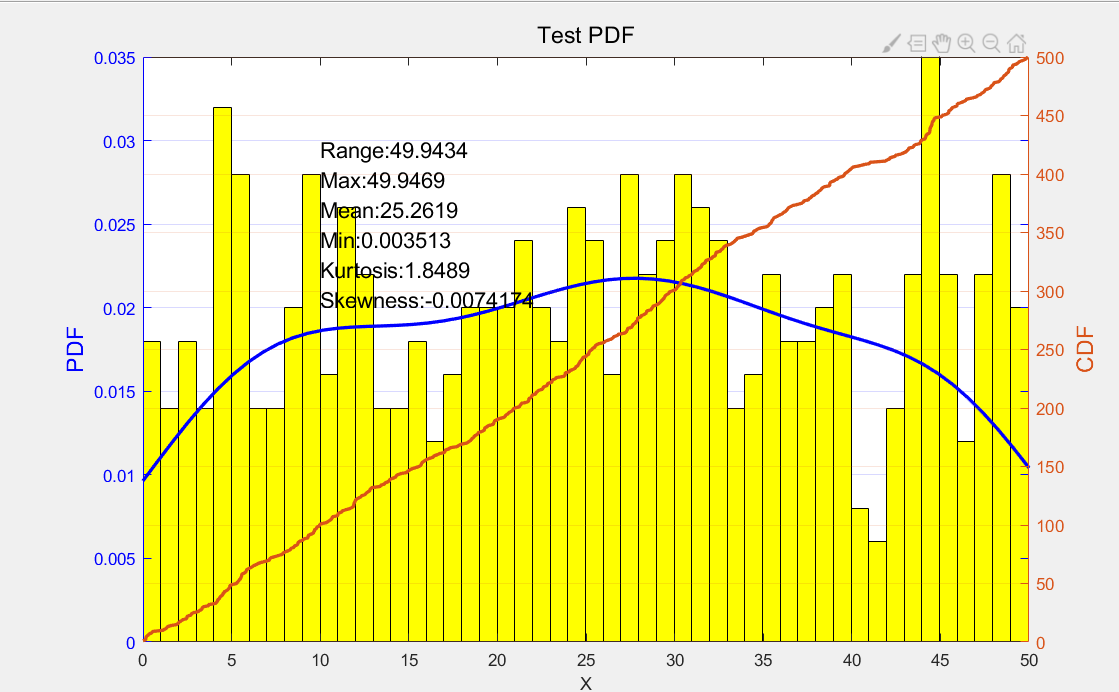注：在编码编写时，可查看已设置的标签属性（必须先运行代码出现图像时，才能显示属性）：
例如：在命令行窗口输入：>>get(AX(2),'ylabel')，可查看对于右侧轴标签的属性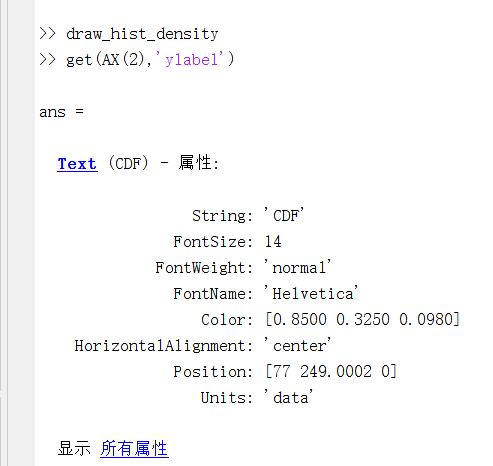展开全文开发语言 统计模型
• X 被定义为在 (0, 1) 上均匀分布的随机变量。 在其他形式中，它会写成 X~Unif(0,1)。 在另一边 Y 是一个新的随机变量，它被定义为 X 的... 之后，估计并绘制 Y 的概率密度函数。找到一个似乎适合估计 PDF 的分析模型。
• 该函数制作归一化直方图，即概率密度函数的估计。 直方图的面积等于 1，因为该面积低于理论 PDF 函数。 您可以使用此脚本的输出将经验数据与特定分布的理论 PDF 进行比较。 输入： 数据 - 经验数据bins - 直方图 ...
• 本文首先给出正态分布概率密度函数（The normal distribution probability density function）的公式和标准正态分布概率密度函数的公式，然后通过normpdf( )生成标准正态... )绘制标准正态分布概率密度函数的图形...
本文首先给出正态分布概率密度函数（The normal distribution probability density function）的公式和标准正态分布概率密度函数的公式，然后通过normpdf( )生成标准正态分布概率密度函数的数据，然后通过plot( )绘制标准正态分布概率密度函数的图形。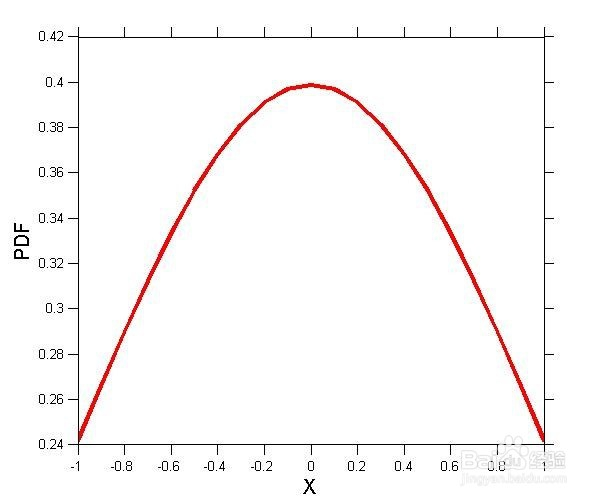工具/原料
MATLAB  normpdf  mean  standard deviation
方法/步骤
第一，正态分布概率密度函数的公式如下图。其中，μ为平均值（mean），σ为标准差（standard deviation）。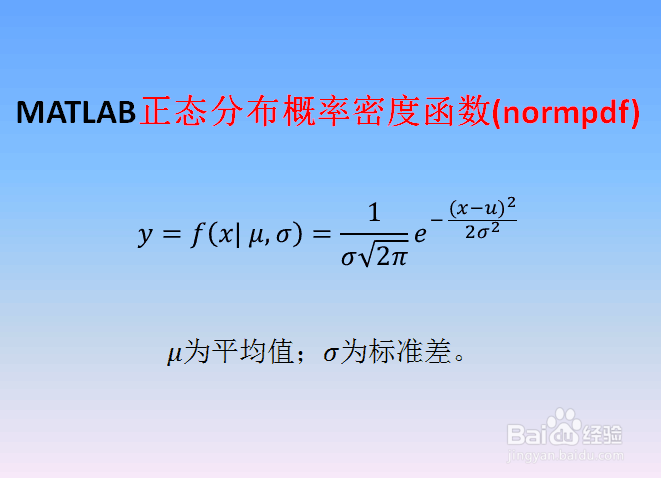第二，当μ=0，σ=1时，第一步中的正态分布为标准正态概率密度函数，如下图。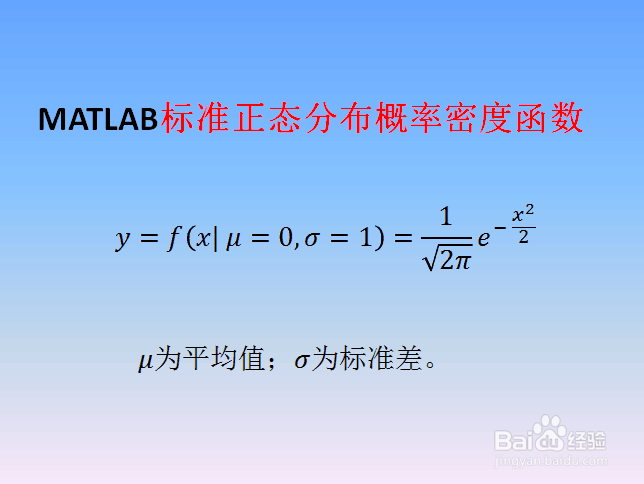第三，启动MATLAB，新建脚本（Ctrl+N），输入如下代码： close all; clear all; clc x=-1:.1:1; norm=normpdf(x,0,1); figure('Position',[50,50,600,500],'Name','Normal PDF',...     'Color',[1,1,1]); plot(x,norm,'r-','LineWidth',3) set(gca,'FontSize',10,'TickDir','out','TickLength',[0.02,0.02]) xlabel('X','FontSize',15);ylabel('PDF','FontSize',15) 其中normpdf(x,0,1)是用来产生μ=0，σ=1的标准正态分布概率密度函数的数据。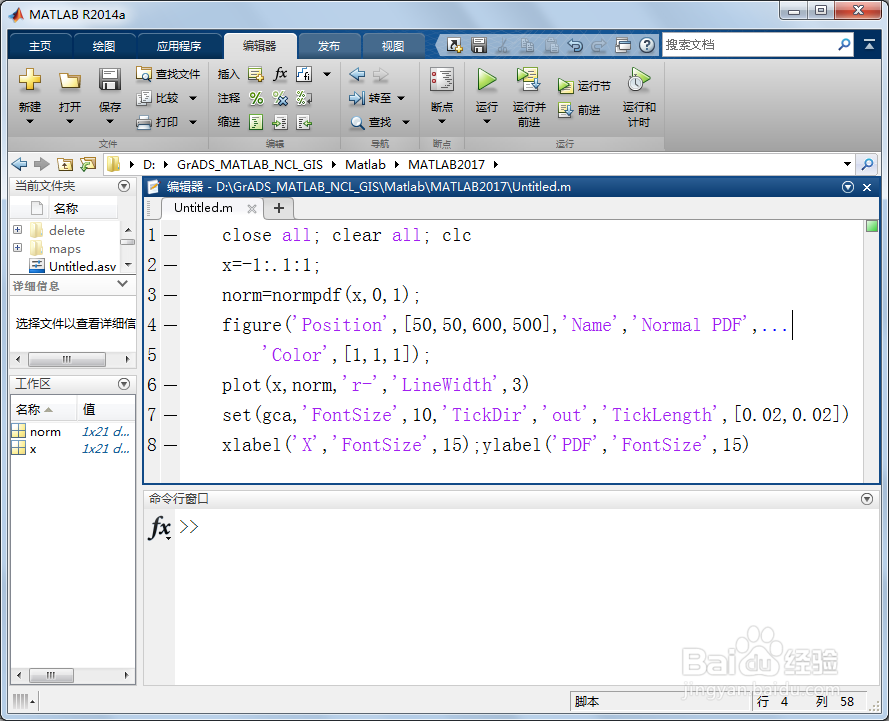第四，保存和运行上述脚本，在工作区（Workspace）得到标准正态分布概率密度函数的数据norm，双击norm可以查看数据具体内容。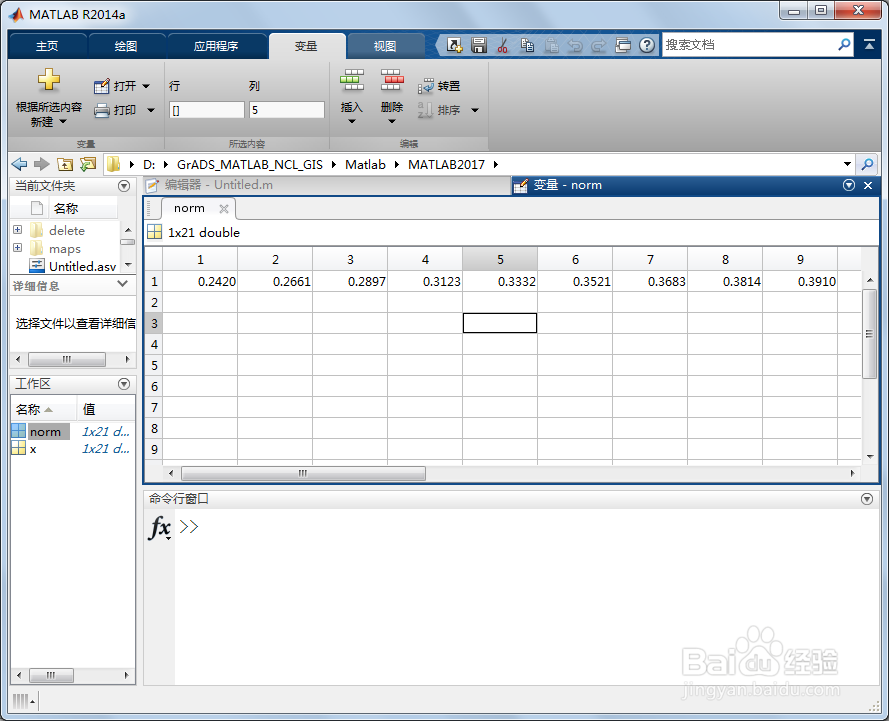第五，同时得到如下μ=0，σ=1的标准正态分布概率密度函数图形。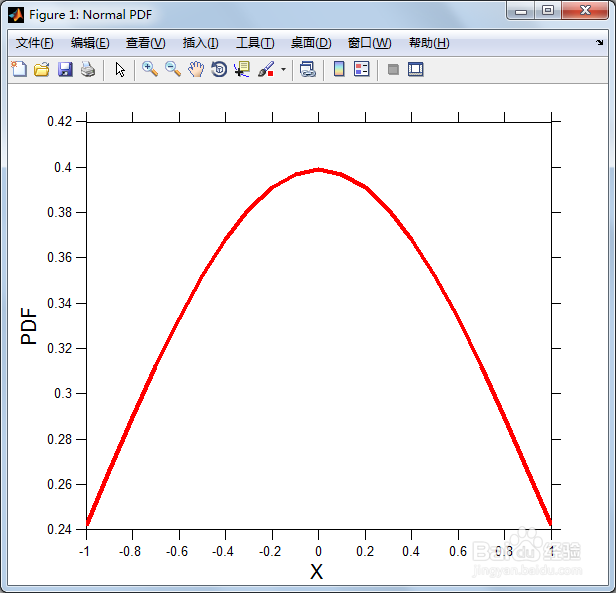展开全文• 这里是一个简单的实现代码 x=linspace（-5,5,50); %生成负五到五之间的五十个数，行矢量 y=normpdf(x,0,1); plot(x,y,‘k’);...第一，正态分布概率密度函数的公式如下图。其中，μ为平均值（mean...
• matlab概率密度函数很简单，但是本人学的python，因此想用python作图，从txt文本中读取数据，然后用python作概率密度函数图像，
• 程序代码： >> clear all >> x=[-10:0.1:10]; >> f1=normpdf(x,0,1); >> f2=normpdf(x,0,2); >> f3=normpdf(x,1,2); >> plot(x,f1,'b*',x,f2,'ro',x,f3,'g+') ...>> legend('X~N(0,1)','X~N(0,4)','X~N(1,4)')
• 概率密度函数 分布概率密度函数（PDF）。 随机变量的（PDF）为 其中v > 0是自由度。 安装 \$ npm install distributions-t-pdf 要在浏览器中使用，请使用 。 用法 var pdf = require ( 'distributions-t-pdf' ) ;...JavaScript
• 二维正态随机变量概率密度函数三维图的matlab实现 1.二维正态随机变量 二维正态随机变量是最常见的一种二维随机变量分布。其联合概率密度函数为： p(x,y)=12πσXσY1−r2⋅exp{−12(1−r2)[(x−mX2)σX2−2r(x−mX)...
• %%一维高斯函数% 生成均值为0，标准差为1的一维高斯概率密度函数 mu=0; sigma=1; x=-6:0.1:6; y=normpdf(x,mu,sigma); plot(x,y); %%二维或多维高斯函数figure;mu=[0 0];sigma=[0.3 0;0 0.35];[x y]=mesh
• 用来画大量样本数据的概率密度曲线，使用简单，快速。数据越多，画的效果越好。
• %密度函数 x1=-1:0.01:4; y1=unifpdf(x1,0,3); %均匀分布 x2=-5:0.001:5; y2=normpdf(x2,0,1); %正态分布 x3=0:0.001:10; y3=exppdf(x3,3); %指数分布 x4=-5:0.01:25; y4=binopdf(x4,100...
• % 绘制概率密度函数% pf为particleFilter函数创建的粒子滤波，修改nbins可调整统计密度particleMax = max(pf.Particles);particleMin = min(pf.Particles);nbins = 50;binWidth = (particleMax-particleMin)/nbins;...粒子滤波
• 使用MATLAB编程对威布尔概率密度函数进行绘制
• 由上面的定理我们可以看出想要用蒙特卡洛方法求三重积分首先要找到一个合适的概率密度函数 ,由上述条件1我们可以知道只要函数 在积分域内的积分为1就行了，其实是存在最有利的概率密度： 其中 。有同学可能看出来了...
• %hist函数用法[区域内数据个数，区域中点值]=hist（样本数据，区域划分） %均为行向量 y_negative=y_negative/length(negative); %将数目化为百分比 y_negative=[(y_negative') (y_negative') (y_negative')]'; y_...开发语言
• MATLAB如何使用chi2pdf函数计算卡方分布的概率密度【语法说明】Y=chi2pdf(X,V)：...【功能介绍】计算卡方分布概率密度函数。卡方分布只有一个参数V，且V为正整数。卡方分布中的随机变量X是V个服从正态分布的随机变...
• ## 高斯概率密度函数

千次阅读 2021-09-18 11:52:56
单变量正态分布概率密度函数定义为： ρ(x)=12πσe−12(x−μσ)2(1) \rho(x)=\frac{1}{\sqrt{2\pi \sigma}} e^{-\frac{1}{2} (\frac{x- \mu}{\sigma})^2} \tag 1 ρ(x)=2πσ​1​e−21​(σx−μ​)2(1) 式中μ\...贝叶斯分类
• 本文档通过MATLAB绘制二维随机变量(X,Y)的联合概率密度函数。其中X服从标准正态分布，Y服从均匀分布。 【例题】已知随机变量X与Y相互独立，X~N(0,1);Y在区间[0,2]上服从均匀分布。求： （1）二维随机变量(X,Y)的...
• 原理也很简单，通过matlab自带的ksdensity获得网格每一点密度，通过密度拟合曲面，再计算每个数据点对应的概率，并将概率映射到颜色即可 为了怕大家找不到函数这次工具函数放到最前面 1工具函数完整代码 function ...
• matlab 二维 正态 概率密度 二维概率分布
• [mu,sigma]=normfit(x); r=normrnd(mu,sigma,1000,1); 根据样本x计算mu和sigma, 使用normrnd生成1000个服从样本概率密度函数的随机数。数据分析
• clear all;...%%一维高斯函数 mu=0; sigma=1; x=-6:0.1:6; y=normpdf(x,mu,sigma); plot(x,y); figure; %%二维或多维高斯函数 mu=[0 0]; sigma=[0.3 0;0 0.35]; [x y]=meshgrid(linspace(-8,8,80)机器学习
• matlab的滤波函数filter与filtfilt比较摘要滤波器应用非常广泛，例如根据位置数据对机器人速度和加速度进行估计时，差分运算会放大噪声，滤波环节是必不可少的。 filter滤波器称为一维数字滤波器。filtfilt滤波器......

# matlab绘制概率密度函数matlab 订阅